## Introduction

In the past decade network science offered deep insights into the structure and dynamics of complex networked systems1,2,3,4,5,6,7. Yet, we continue to lack tools to efficiently control the dynamics of complex networks. According to control theory8,9,10, a dynamic system is controllable if suitable external inputs can move the internal state of the system from any initial state to an arbitrary accessible final state in a finite time interval11. Recently, we introduced an analytical framework to study the controllability of complex networks via a combination of tools from network science, control theory and statistical physics12. By mapping the structural controllability problem13 to a maximum matching problem14, we were able to identify a minimum set of driver nodes of size ND, sufficient to control the whole network12,15. For engineered systems, such as the auto-pilot system of an airplane, full control is essential. However, many biological, technological and social systems are massive in size and complexity, hence it is neither feasible nor necessary to control the full network. Instead, it is more realistic and sufficient to achieve target control, that is, to control a subset of target nodes (or a subsystem) that are essential for the system’s mission pertaining to a selected task. While potential applications of target control have been developed in domain-specific areas in biology16, chemical engineering17, epidemics18 and economic networks19, a principled approach to identify a minimum set of driver nodes sufficient for the targeted control of an arbitrary complex network remains an open problem.

We study two distinct schemes for choosing the subset of nodes we wish to control. In the random scheme, a fraction f of nodes are chosen uniformly at random. In the local scheme, the chosen nodes form a connected component, capturing a well-defined local network neighbourhood. To develop an efficiency measure of target control, we compare PD, which is the minimum number of driver nodes needed to control the fraction f of target nodes, with fND, which is the corresponding relative fraction of the driver nodes needed for full control. If PD is less than fND then target control is more efficient than full control for a given f. We also establish the overall target control efficiency by considering the integrated efficiency across the entire range of 0<f≤1. For both scale-free (SF)20 and Erdös–Rényi (ER) networks21,22, we find that in general, local target control is more efficient than random target control. More surprisingly, we find that degree heterogeneous networks, such as SF networks, have higher specific and overall target control efficiency than degree homogeneous networks, for both random and local schemes, when the average degree of the network is large. In contrast, for full control, degree homogeneous networks, such as ER networks, are more efficient than the corresponding degree heterogeneous networks12. Finally, we apply our methods to real data, confirming that many real-world networks display high efficiency of target control.

## Results

### Model

The dynamics of most real systems is driven by nonlinear processes. However, the dynamical rules of real-world networks are so diverse that writing a general dynamical equation that captures them all is plainly impossible. Moreover, for many networks, especially biological networks, we do not even know the dynamical rules. Thus, before we explore the fully nonlinear dynamical problem, we have to understand the impact of the topological characteristics on linear control, which naturally serves as a prerequisite of the nonlinear controllability problem. Furthermore, the controllability of nonlinear systems is often structurally similar to and determined by the system’s linearized dynamics23. Indeed, a basic starting point for exploring the controllability of any nonlinear system is the study of the linearized version of the nonlinear dynamical system. Therefore, we start with the canonical linear time-invariant dynamics.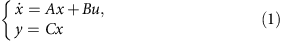where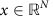,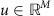and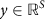represent the system’s state, input and output vector, respectively.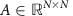,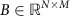and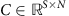denote the state, input and output matrices, respectively. A captures the wiring diagram of the system; B identifies the nodes that are controlled by an external controller and u is the time-dependent input applied to the nodes in B; C is the output matrix identifying the target nodes we want to control. For a network with node set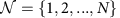, we are interested in controlling a target node set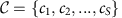of size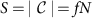. We set the output matrix C=[I(c1),I(c2),... I(cS)], where I(i) denotes the ith row of an N × N identity matrix I. The system (A, B, C) is said to be target controllable with respect to a given target node set C if there exists a time-dependent input vector u(t)=(u1(t),... uM(t))T that can drive the state of the target nodes to any desired final state in finite time. Target controllability can be viewed as a special type of output controllability and the system (A, B, C) is target controllable if and only if the dimension of the output controllable subspace d(A, B, C) satisfies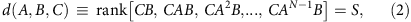representing the mathematical condition for target controllability24. Note that when S=N, C is the identity matrix and (2) reduces to the Kalman condition for full controllability11. If (2) is satisfied, for initial condition x(0)=0, we can compute the optimal input vector u(t) such that we can reach the desired final state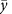with minimum energy cost in time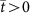(that is,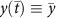) (ref. 24).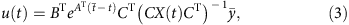where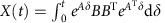(Supplementary Note 1).

In some ways the target control problem is more difficult than the full control problem. Full control has a graphical condition, which can be easily checked by exactly mapping the controllability problem to the maximum matching problem. Target control lacks such an exact mapping. Therefore, to solve the target control problem in the single-input case, we develop a new approach that we call the ‘k-walk theory’ (Fig. 1; Supplementary Fig. 1; Supplementary Note 2). This theory is based on the principle that a node can control a target set of nodes provided that the length of the path from the control node to each target node in the set is unique. Using the k-walk theory, we can identify all sets of nodes that can be controlled by the given node. We show that the k-walk theory is more powerful than the standard approach as it can find controllable subsystems that the structural control theory misses (Fig. 1; Supplementary Note 2). We rigorously prove that the k-walk theory is correct for directed tree-like networks, that is, networks with no loops (Supplementary Note 2).Figure 1: Structural control theory versus the k-walk theory for target control.

Although powerful, the k-walk theory is only applicable for the single-input case. For networks that require >1 control input, we formulate the target control problem in graph theoretic terms, allowing us to develop a greedy algorithm (GA) that offers a good approximation to the minimum set of inputs sufficient for target control (Fig. 2). We rigorously prove that the input set selected by our algorithm can indeed control all target nodes (Fig. 3; Supplementary Note 3). Both the k-walk theory and the GA are based on the structural control theory, that is, the system parameters are either fixed at zero or are independent free parameters. This approach has lead to several recent advances on network control19,25,26,27,28,29,30,31,32,33,34. Structural control theory, in general, relies on the canonical linear time-invariant dynamics of the system as discussed in the Methods.Figure 3: Full versus target control of the simple network shown in Fig. 1.

Previous studies based on the structural control theory shed some light on how to determine the upper bound of the minimum number of driver nodes for target controllability35,36. We can use the maximum matching method to find the minimum set of driver nodes (ND nodes) for full control of the network (Fig. 2a–c). This results in ND disjoint ‘cacti’ (Supplementary Note 3), each denoting the control region of a particular driver node under one specific maximum matching. We can then count the minimal number of disjoint cacti that cover all target nodes, which yields an upper bound of PD for target control (denoted as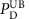) (see Fig. 2; Supplementary Note 3 for details). The lower bound of PD, denoted with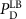, can also be obtained using maximum matching. We consider the maximum matching in a bipartite graph connecting on one side (1) the target nodes (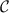nodes), and on the other (2) the nodes that can reach the target nodes via (3) the edges among them. If a target node is unmatched, we must drive it directly, and the number of unmatched target nodes is the lower bound of PD. Taking Fig. 2 for example, the lower bound of PD is given by the first iteration of the GA, that is,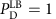for the target nodes {1, 2, 4, 6, 7}, because in the first iteration only node 1 is unmatched.

### Target controllability

To quantify the efficiency of target control for a specific fraction f, we define the target controllability parameter αDPD/ND (Methods section) and investigate it for both random and local control schemes, as shown in Fig. 4a,d, respectively. Note that for f=1, we have αD=1 as target control reduces to full control. In Fig. 4 the black lines denote the neutral condition (αD=f) and serve as a benchmark because they mean that controlling an f fraction of target nodes requires an f fraction of the driver nodes needed for full control, that is, PD=fND. In each figure, UB denotes the upper bound of the minimum number of driver nodes needed to control an f fraction of target nodes, predicted by the structural control theory (Supplementary Note 3). Likewise, GA denotes the results of the greedy algorithm and LB (Supplementary Note 3) denotes the lower bound, which is obtained by the first step of the GA (Methods section). Figure 4b shows the results of random target control of ER networks. Here the GA curve is above the neutral line (αD>f), which indicates that the target control is less efficient than the neutral expectation. Figure 4c shows the results of random target control on SF networks and the GA curve is almost at the neutral line (αDf), which indicates that target control is as efficient as the neutral expectation. If we apply the local target control scheme to an ER network, we observe reduced efficiency for f<0.5 (minus symbol in Fig. 4e) and enhanced efficiency for f>0.5 (plus symbol in Fig. 4e). Figure 4f shows the case of local target control on SF networks, charactering that the target control is more efficient (that is, αD<f).Figure 4: Random versus local target controllability of two canonical network models.

In general, we find that the local target control scheme requires fewer driver nodes when compared with the random target control scheme. This can be explained as follows. The GA helps us obtain an approximately minimal set of driver nodes sufficient to control the target nodes. Yet, the size of the controllable subsystem (or equivalently, the dimension of the controllable subspace) can be larger than the size of the target node set. In other words, we may actually be able to control a larger subsystem than necessary.

In Supplementary Fig. 3, we show that the random target control scheme has larger controllable subsystems than the local target control scheme. This is true for any fraction f of target nodes. Hence the local control scheme is more efficient than the random control scheme. Furthermore, we find that αD is robust to changes in network size as shown in Supplementary Fig. 6. Finally, since the hubs play an important role in complex networks, we study the case of controlling an f fraction of the highest in- and out-degree nodes as target nodes (Supplementary Fig. 5). In general, we find that controlling the high-degree nodes reduces the minimum number of driver nodes.

### Target control efficiency

The above observations raise a fundamental question: what kind of network topology is more efficient for target control and which control scheme (random or local) facilitates target control for a general f fraction of target nodes? To address this question, we define the overall target control efficiency in the Methods. The overall target control efficiency of two model networks (ER and SF) are shown in Fig. 5 as provided by simulations. When ‹k›=0 (a network with N isolated nodes), we have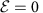. That is, if we wish to control an f fraction of nodes we need to drive the same fraction of driver nodes as in the case of full control. We observe a peak of target control efficiency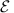for SF networks with degree exponent γε[2, 3], which is the range of γ relevant for many real-world networks37,38 (Fig. 5a,c).

This optimal target control efficiency is likely due to the presence of the hubs. Indeed, starting from an ER network, we can fix the number of links and rewire the network to increase the degree of a preselected node, turning it into a hub. We find that the target control efficiency increases as we develop a hub (Supplementary Fig. 4; Supplementary Note 4), explaining the increase of efficiency as we lower γ from γ>3 to γ≈2.5.

Overall, Fig. 5a suggests that many real-world networks may have been optimized for efficient target controllability. Interestingly, we find that networks, which are easier to control if we wish to achieve full control, that is, those with large average degree ‹k› or large degree exponent γ12, do not show high efficiency for target control. Moreover, SF networks with 2≤γ≤3, which are harder to fully control than ER networks, have high random target control efficiency (Fig. 5c). Compared with ER networks, a SF network has lower local target control efficiency when the average degree ‹k› is small but higher local target control efficiency when the average degree ‹k› is large (Fig. 5d). Figure 5d also indicates the existence of a critical ‹kc so that when ‹k›>‹kc SF networks are more efficient than ER networks for target control. In general, sparse and homogeneous networks display high efficiency for target control, especially in the local scheme.

### Target control of real networks

Next we apply the tools developed above to several real networks divided into seven categories, which are chosen for their diversity in applications and scope (Fig. 6; Table 1). We find that networks from the same category display similar target control efficiency (Fig. 6a,d). Interestingly, only networks with large average degree (‹k›>‹kc>2.4) are more efficient than their randomized counterparts with respect to the local target control scheme, that is,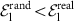(with values indicated in italics in Table 1, column ‹k› and in Fig. 6f). This is in agreement with simulations (Fig. 5d). We observe that local target control markedly increases the control efficiency of neuronal networks (Fig. 6e). The opposite result is obtained for the power grid, finding that random target control is more efficient than the local control (Fig. 6b,e). This implies that the structure of the power grid is not optimized to facilitate local target control. For all gene networks target control efficiency is close to 0, indicating that they have evolved towards a topology that has comparable control efficiency within any sub-segment. Also, in the random target control scheme, the comparison between real networks and randomized networks support our earlier conclusion: real networks are optimized to have high random target control efficiency, because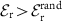for almost all of them. The two counter-examples are the trust networks, which are extremely small, however, with only 32 and 67 nodes. The target control efficiency of real networks is summarized in Table 1.

## Discussion

In this work, we studied the target controllability of complex networks. We developed a new theoretical approach, the k-walk theory, to identify the controllable sub-graph that one node can control, and a GA to identify an approximately minimum set of driver nodes to control a specified target set of nodes. We studied both random and local target control schemes and analysed how the network topology impacts the target control efficiency. The GA proposed in this work is efficient in finding the driver nodes for target control when the network structure is completely known. In reality, we have very incomplete maps of many real-world networks and the full controllability of networks with missing links has been recently addressed in ref. 39. Note that if we add the missing links back, they will only enhance the target controllability of the system, unless the link weights satisfy some particular algebraic constraints, which is a typical zero-measure event.

Our results raise several open questions: what higher-order network characteristics (such as communities, degree correlations) determine target controllability and target control efficiency? How to design the control inputs to steer the target nodes towards a desired final state? How to apply the GA to the study the target control of link dynamics?

Finally, as discussed in the Methods section, our formulation considers the canonical linear time-invariant dynamics of a system. Extending these findings to networks with fully nonlinear dynamics may require connecting our current work with complimentary approaches that consider the basins of attraction that describe the steady-state properties of nonlinear dynamics10,40. Understanding these questions can significantly improve our understanding of the control principles of complex systems.

## Methods

### Greedy algorithm

The GA we introduce here is based, in part, on iterating the procedure for the lower-bound calculation to ultimately approximate the minimum set of driver nodes (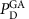) for target control (Fig. 2d,e). The lower-bound of PD can be calculated as follows: (1) Construct a bipartite graph , where the right side consists of all the target nodes, and the left side consists of all the nodes that can reach the target nodes. There is a link between node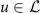and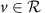if there is a link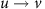in the original directed network . (2) Find a maximum matching in . The number of unmatched nodes in yields the lower-bound of PD. The greedy algorithm works as follows. (1) Initialize the setto be the set of the unmatched nodes found from the lower-bound calculation. (2) Identify the set of nodes in that match the nodes in , and let this node set to be the new set and get a new bipartite graph. (3) Calculate a maximum matching in the updated bipartite graph and add unmatched nodes in to the set. (4) Repeat (2) and (3) until all nodes have been matched or are in the set. In Fig. 2d,e, we offer a specific example and the details of the GA. The proof of its sufficiency for target controllability is provided in Supplementary Fig. 2 and Supplementary Note 2. Figure 2e can also be obtained by the k-walk theory, because the length of the path from the node 1 to each target node {1, 2, 4, 6, 7} is unique. Note that if the GA converges after only one iteration, we obtain the exact number for the minimum number of driver nodes for target control.

A direct illustration of our algorithm’s utility is illustrated in Fig. 3, where we consider control of the network shown in Fig. 2. To control the entire network, we need at least three driver nodes. Indeed, as we show in Fig. 3a,b using these three nodes we can move the state of all nodes to the desired final state xi=10. But if we just want to control a subsystem {1, 2, 4, 6, 7} (highlighted in red in Fig. 2e), the GA predicts that we need a single driver node, node 1. Indeed, as we show in Fig. 3c,d we can now move the state of the target nodes to the desired final state through an input to node 1 only. The nodes outside of the target list take arbitrary values as we do not control them.

### Target control efficiency

We define the overall target control efficiency of an arbitrary network as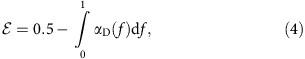denoting the efficiency of random and local target control scheme by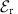and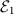, respectively. For example, forthe overall network efficiency is neutral, that is, to control an f fraction of target nodes we need fND driver nodes. When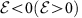, the network is less (or more) efficient than neutral expectation. Furthermore,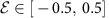, so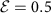corresponds to the most efficient case and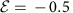shows the least efficient case. One example of the least efficient case is when only one driver node is needed to control the whole network. If we control any fraction of nodes, we still need one driver node, thus(observed for Food Web and Neuronal networks in Table 1). Note that target controllability for a specific fraction, αD(f), depends on the fraction f of driver nodes, but overall target control efficiency,, is a property of the whole network, independent of the fraction of target nodes.